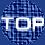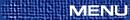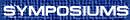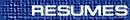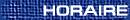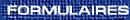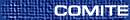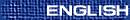Dynamical Systems / Systèmes dynamiques

BHAGWAN AGGARWALA, University of Calgary, Calgary, Alberta
Application of predator prey equations to development of HIV/AIDS in Canada

We consider the ratio dependent predator-prey equations of mathematical biology and watch the very rich behavior of solutions unfold as the conversion factor changes. For large values of the conversion factor, we encounter repeated bifurcations and stable and unstable limit cycles. These limit cycles disappear as this parameter is further increased. We apply these equations to the spread of HIV/AIDS in the Canadian society and notice that unless the number of healthy people in the society begins to grow much faster than at present moment, we are in for hard times!

WILLIAM BASENER, Rochester Institute of Technology
Disk global cross sections in flows on manifolds

For a flow on a manifold, a global cross section is an imbedded co-dimension 1 manifold which intersects the orbit through every point in positive and negative time. Global cross sections without boundary are a standard tool which capture global topological properties of a flow. We show that global cross sections that are a disk capture many of the same properties of the flow. We will prove that, unlike global cross sections without boundary, every continuous flow on a manifold of dimension greater than 2 has a global cross section that is a disk.

BERNARD BROOKS, Rochester Institute of Technology
A rrequency and density dependent multi-allele haploid dynamic

A discrete model of frequency and density dependent haploid evolution will be presented along with the conditions for the linear stability of the equilibria of the dynamical system. The organisms in question are multi-allele and simulations of the results will be shown.

PIETRO-LUCIANO BUONO, CRM, Universite de Montreal, Montreal, Quebec  H3C 3J7
Restrictions on flows in double Hopf bifurcations for functional differential equations

I am going to discuss the normal form of vector fields generated by delay differential equations at double Hopf bifurcation points. The main results presented will be:

1)  there are generically no restrictions on the possible flows of scalar first order equations with two delays in the nonlinearity,

2)  There are always restrictions on the possible flows for scalar first order equations with one delay in the nonlinearity and n-th order delay equations (n ³ 2) with one delay in the feedback. These results are based on computations of normal form for delay differential equations via the method of Faria and Magalhaes.

MONICA GABRIELA COJOCARU, Queen's University
Projected dynamical systems

We present a dynamical system constrained to a closed, convex subset of a Hilbert space of arbitrary dimension. It is a generalization of a notion introduced in the Euclidean space. A projected dynamical system is defined by the solutions of a differential equation with a discontinuous and nonlinear right-hand side. We will outline the proof of existence of such systems and emphasize the difficulties encountered and the techniques we used.

ABBA GUMEL, University of Manitoba
Dynamics analysis of the effect of condom use on HIV epidemiology

(joint work with S. M. Moghadas and R. Gordon (University of Manitoba).)

There are two major approaches for controlling the HIV epidemic namely ``prevention'' and therapeutic treatment. Although the new anti-HIV therapies appear promising, prevention remains the most effective weapon against the epidemic. This paper studies the effect of condom use in HIV prevention in the absence of any treatment. A new deterministic model, for monitoring the community-wide effect of condom use, is designed and analyzed qualitatively. Based on the stability and numerical analyses of the model, our study shows that community-wide eradication of HIV is feasible provided the condom ``preventability'' parameter, defined as the product of condom efficacy and compliance in its use, exceeds a certain threshold.

HENRIK KALISCH, McMaster University
The effect of attenuation and amplification on dispersion-managed solitons

The dynamic properties of pulses in a dispersion-managed optical fiber transmission system are investigated using a reduction to a two-dimensional system of ordinary differential equations based on a Gaussian Approximation. In particular, pulses which are nearly stationary are investigated in regard to stability and their behavior with respect to limits of certain system parameters.

CANDACE KENT, Virginia Commonwealth University
The boundedness nature of positive solutions of the sifference squation x[n+1] = max{ A[n]/x[n], B[n]/x[n-1]} with periodic parameters

We investigate the boundedness nature of positive solutions of the difference equation

 x[n+1] = max { A[n]/x[n], B[n]/x[n-1] },     n=0,1,...,
where {A[n]} is a periodic sequence of positive numbers with prime period p and {B[n]} is a periodic sequence of positive numbers with prime period q. We will give necessary and sufficient conditions for every positive solution to be unbounded.

WITOLD KOSMALA, Appalachian State University
On the recursive sequence x(n+1) = (p + x(n-k))/x(n)

We investigate the periodic character, boundedness, stability of solutions, and properties of semi-cycles of the nonlinear difference equation

 x(n+1) = æè p + x(n-k) öø /x(n)
where the parameter p and the initial conditions are positive real numbers. Joint work together with Elias Camouzis and Richard DeVault.

HERBERT KUNZE, University of Guelph
Solving inverse problems in differential equations using Banach's fixed point theorem

Banach's fixed point theorem plays a role in the (often approximate) solution of many inverse problems in mathematics. The essential idea is to express the problem in terms of a contractive map on a complete metric space in order to use the fixed point theorem and some more recently developed related results, most notably Barnsley's collage theorem. For the inverse problem, one is given a solution, possibly an approximation or an interpolation of experimental data, and then one seeks a map of a particular form which has this ``target'' solution as a fixed point. In this talk, we consider the problem: Given a function x(t), possibly an interpolation of data points, with t Î [0,1], find an ODE x¢=f(x,t), x(0)=x0, that admits x(t) as a solution as closely as desired, where f is restricted to a class of functional forms (affine, quadratic in x, etc.). A collage-like theorem is established, with the end result being an algorithm which produces a least-squares minimization problem. Many of the parameter estimation techniques used by researchers in differential equations can be viewed as inverse problems of the outlined type; this work appears to be the first rigorous mathematical justification of such ad hoc methods of error minimization.

WILLIAM LANGFORD, University of Guelph
Near-reversible 1:1 resonant Hopf bifurcation

The standard Hopf bifurcation theorem does not apply to reversible vector fields, but is replaced by the ``reversible Hopf bifurcation theorem'' in which two pairs of complex conjugate eigenvalues approach the imaginary axis symmetrically from the left and right, then merge in double purely imaginary eigenvalues and separate along the imaginary axis. The merging is called 1:1 resonance, and has codimension one in the class of reversible vector fields. In the general case of non-reversible vector fields, occurrence of 1:1 resonant double imaginary eigenvalues has codimension three. This talk will present an investigation of the interface between the reversible and non-reversible 1:1 resonant Hopf bifurcations, which we call the near-reversible case. The investigation proceeds in both directions; first as a restriction of the general case of codimension three 1:1 resonance, and second as a dissipative perturbation of the reversible case taking advantage of the first integrals that exist there. We show that interesting bifurcation phenomena known to exist in the general non-reversible case persist in the near-reversible case, and we reveal some new phenomena.

This is joint work with G. Iooss, INLN-CNRS, France.

ISRAEL NCUBE, York University
Possible change in criticality of Hopf bifurcation in a multiple-delayed neural system

We consider a network of three multiple-delayed identical neurons, consisting of a single discrete delay and a continuously distributed delay. The model for such a network is a system of delay differential equations (DDEs). It is shown that two cases of a single Hopf bifurcation may occur at the trivial fixed point of the full nonlinear system of DDEs. These are (i)  the simple root Hopf, and (ii)  the double root Hopf. This presentation focusses on (ii), with particular attention paid to the mechanics (and problems) of deriving the corresponding system of ordinary differential equations describing the semiflow on the centre manifold. Our long term objective is to characterise the criticality of the single Hopf double root case.

DMITRY PELINOVSKY, McMaster University
Spectral analysis of optical bistability in finite and semi-infinite nonlinear photonic gratings

We study optical bistability in nonlinear periodic structures of finite and semi-infinite length. For finite-length structures, the system exhibits instability mechanisms typical for dissipative dynamical systems like delay-differential equations. We show that the Leray-Schauder degree equals the sign of the Evans function in l = 0. Using the Evans function methods, we rigorously prove that the stationary solutions with optically bistable transmission characteristics are indeed spectrally unstable. For semi-infinite structures, the system exhibits two pulse solutions, when the infinite Hamiltonian dynamical system has soliton solutions, and one pulse solution, otherwise. We show that the non-monotonic pulse solution is always spectrally unstable. Numerical computations of the linear stability problem and time-dependent coupled-mode equations confirm the analytical results.

In time-dependent dynamics, the incident light waves are uniformly stable and all-optical limited if the photonic grating has zero net-average Kerr nonlinearity. When the average nonlinearity is weak but non-zero, light waves exhibit oscillatory instabilities and long-term high-amplitude oscillations in the out-of-phase linear gratings. We show that a two-step transmission map between lower-transmissive and higher-transmissive states has a narrow stability domain, which limits its applicability for logic and switching functions. Light waves exhibit cascades of real and complex instabilities in the multistable gratings with strong net-average Kerr nonlinearity. Only the first lower-transmissive stationary state can be stimulated by the incident light of small intensities. Light waves of moderate and large intensities are essentially non-stationary in the multistable gratings and they exhibit periodic generation of Bragg solitons and blow-up.

MARIO ROY, Concordia University
Is the composition of two expansive maps expansive?

We will investigate the question whether the composition of two (positively) expansive maps is itself expansive. Two mutually opposed situations will confront us. On the one hand, recall that the composition of two maps that are expanding with respect to the same metric is expanding. On the other hand, the composition of two expansive homeomorphisms is generally not expansive (for instance, consider an expansive homeomorphism and its inverse). Which of these two situations will ``prevail''?

SHIGUI RUAN, Dalhousie University
Dynamical behavior of an epidemic model with a nonlinear incidence rate

In this paper we study the global dynamics of an epidemic model with vital dynamics and nonlinear incidence rate of saturated mass action. By carrying out global qualitative and bifuraction analyses, it is shown that either the number of infective individuals tends to zero as time evolves or there is a region such that the disease will be persistent if the initial position lies in the region and the disease will disappear if the initial position lies outside this region. When such a region exists, it is shown that the model undergoes a Bogdanov-Takens bifurcation, i.e., it exhibits a saddle-node bifurcation, Hopf bifurcations, and a homoclinic bifurcation. Existence of none, one or two limit cycles is also discussed.

HASSAN SEDAGHAT, Department of Mathematics, Virginia Commonwealth University Richmond, Virginia  23284-2014, USA
On semiconjugates of one dimensional maps

R-semiconjugates maps are defined as a natural means of relating a map F of the m-dimensional Euclidean space to a mapping g of the interval via a link map H. Invariants are seen to be special cases of semiconjugate links where g is the identity. Basic relationships between the dynamical behaviors of g and F, and conditions under which a link map H is a Liapunov function are outlined. Examples and applications involving concepts from stability to chaos are discussed.

MICHAEL YAMPOLSKY, University of Toronto